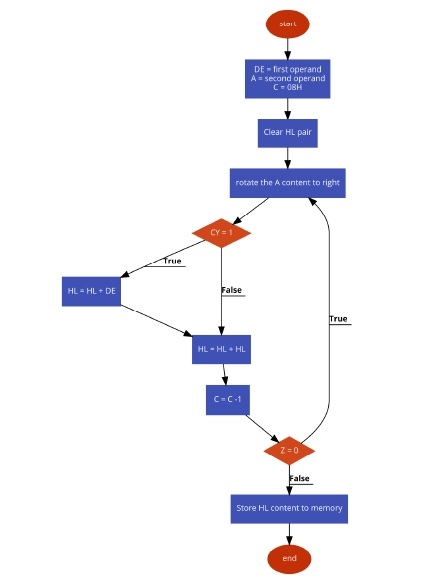Program to multiply two 8-bit numbers (shift and add method) in 8085 Microprocessor

Let us see one 8085 Microprocessor problem. In this problem we will see how to multiply two numbers using shift and add methods, not by using additive approach.

Problem Statement

Write an 8085 Assembly language program to multiply two 8-bit numbers using shift and add method.

Discussion

The shift and add method is an efficient process. In this program, we are taking the numbers from memory location 8000H and 8001H. The 16 bit results are storing into location 8050H onwards.

In this method we are putting the first number into DE register pair. The actual number is placed at E register, and D is holding 00H. The second number is taken into A. As the numbers are 8-bit numbers, then we are shifting the Accumulator contents eight times. When the carry flag is set while rotating, then the DE content is added with HL. Initially HL pair will hold 0000H. Then HL is also added with HL itself. Thus the result will be generated.

Data

8000
25
8001
2A

Flow DiagramProgram

HEX Codes
Labels
Mnemonics
F000
21, 00, 80

LXI H,8000H
Point to first operand
F003
5E

MOV E,M
Load the first operand to E
F004
16, 00

MVI D,00H
Clear the register D
F006
23

INX H
Point to next location
F007
7E

MOV A,M
Get the next operand
F008
0E, 08

MVI C,08H
Initialize counter with 08H
F00A
21, 00, 00

LXI H, 0000H
Clear the HL pair
F00D
0F
LOOP
RRC
Rotate the acc content to right
F00E
D2, 12, F0

JNC SKIP
If carry flag is 0, jump to skip
F011
19

Add DE with HL
F012
EB
SKIP
XCHG
Exchange DE and HL
F013
29

Add HL with HL itself
F014
EB

XCHG
Exchange again the contents of DE and HL
F015
0D

DCR C
Decrease C register
F016
C2, 0D, F0

JNZ LOOP
if Z = 0, jump to LOOP
F019
22, 50, 80

SHLD 8050H
Store the result
F01C
76

HLT
Terminate the program